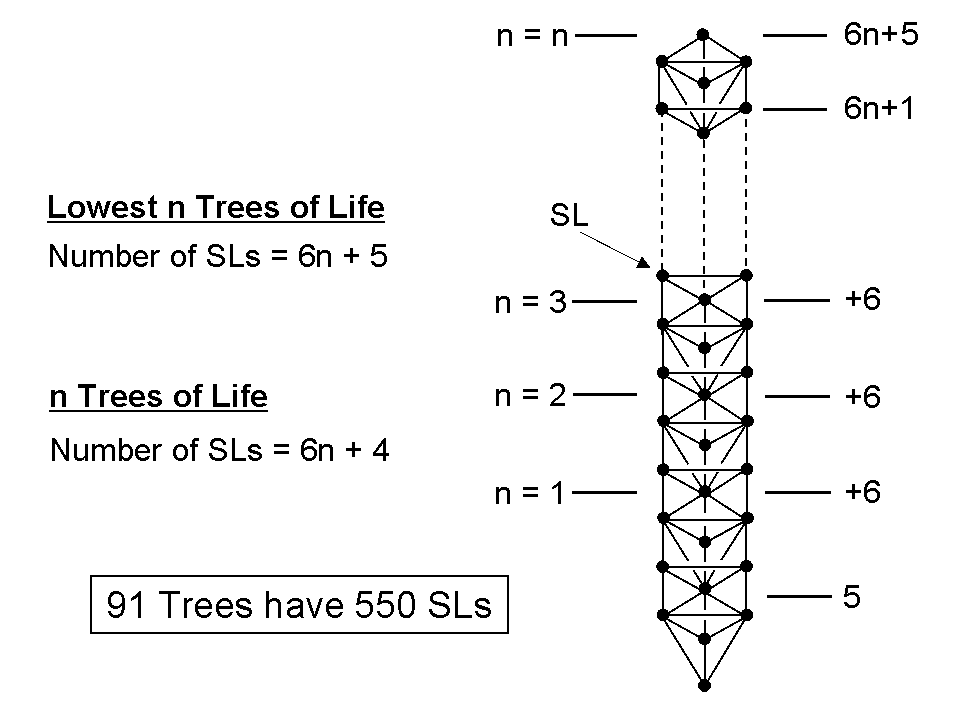Search

# tree of life superstring theory part 33

Updated: Sep 27, 2020The ten yods in the tetractys symbolize the ten Sephiroth, each of which can be represented by a Tree of Life. Suppose, then, that we regard as a Tree of Life each of the 91 triangles (all 'yods' of tetractys arrays of ten triangles) in the Cosmic Tetractys representing differentiations of the Sephiroth of Construction. How many Sephirothic levels, or 'SLs,' are there in the 91 overlapping Trees of Life? The formula for the number N(n) of SLs in n overlapping Trees of Life is*:

N(n) = 6n + 4.

Therefore, N(91) = 550. As will be proved in this section, the number 550 is embodied in all sacred geometries because they are equivalent maps of all levels of reality — physical and superphysical. The set of 91 overlapping Trees with 550 SLs (see opposite) will be called the "Cosmic Tree of Life," or "CTOL." This ladder-like structure is the basis of what is referred in the Old Testament as "Jacob's ladder" (see Book of Genesis 28: 10-19).

* Proof: Consider n overlapping Trees of Life. Above Malkuth, Yesod, Hod & Netzach of the lowest Tree are six more SLs up to (but not including) Kether of this Tree. Each successive, higher Tree adds six SLs. The number of SLs below the top of the (n−1)-tree = 4 + 6(n−1) = 6n − 2. There remain six SLs up to Kether of the nth Tree. Hence, the number of SLs in n overlapping Trees = 6n − 2 + 6 = 6n + 4.